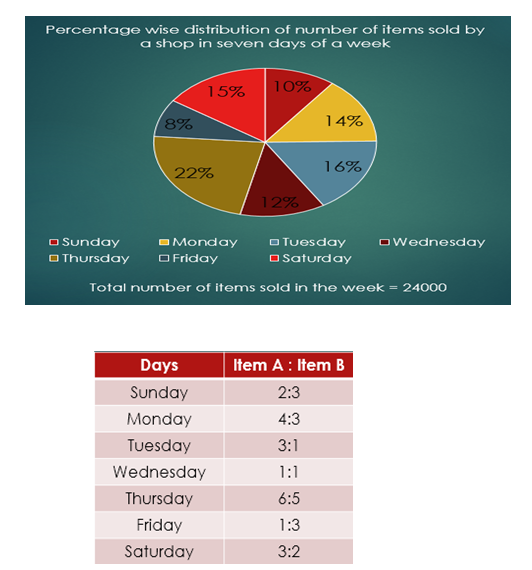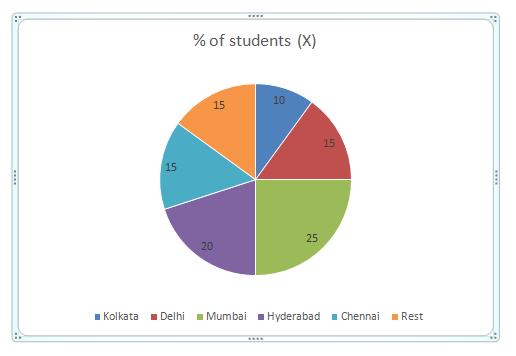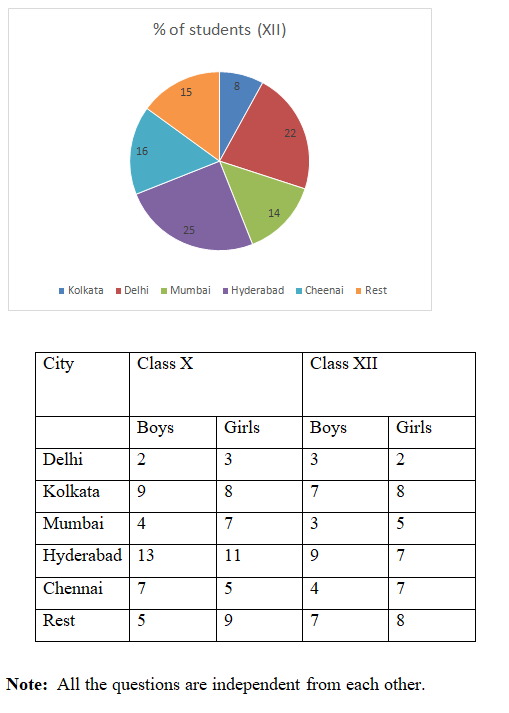# Quantitative Aptitude Questions (Data Interpretation) for SBI Clerk / IDBI Executive 2018 Day-72

Dear Readers, SBI is conducting Online preliminary Examination for the recruitment of Clerical Cadre. preliminary Examination of SBI Clerk was scheduled from June 2018. To enrich your preparation here we have providing new series of Data Interpretation – Quantitative Aptitude Questions. Candidates those who are appearing in SBI Clerk Prelims and IDBI Executive Exams can practice these Quantitative Aptitude average questions daily and make your preparation effective.

[WpProQuiz 2022]

Click “Start Quiz” to attend these Questions and view Solutions

Directions (Q. 1 – 5): Study the following information carefully and answer the questions given below:1. Total number of items sold in Sunday, Monday and Tuesday together is approximately what percent of the total number of items sold in Wednesday, Thursday and Friday together?

a) 91 %

b) 95 %

c) 85 %

d) 88 %

e) 81 %

1. Total number of item A sold in Monday and Tuesday together is what percent more than the total number of item B sold in Friday and Saturday together?

a) 61.12 %

b) 66.67 %

c) 56.78 %

d) 52.46 %

e) 71.34 %

1. Find the respective ratio of total number of item A sold in Thursday, Friday and Saturday together and total number of item B sold in Monday, Tuesday and Wednesday together.

a) 17:21

b) 23:11

c) 23:16

d) 15:37

e) None of these

4. Total number of item B sold in Tuesday, Wednesday and Thursday together is what percent of the total number of items sold throughout the week?

a) 20%

b) 30%

c) 25%

d) 15%

e) None of these

1. Find the total number of item A sold throughout the week.

a) 16420

b) 14520

c) 12720

d) 13750

e) None of these

Directions (Q. 6 – 10) Read the following information carefully and answer the following questions carefully. The graphs below shows percentage of students (Boys + Girls) appeared from different cities in class X and XII examination. The Table shows the ratio between the boys and girls from different cities.1. In class X exam, 280 girls appeared from Mumbai then find the average number of students appeared from Kolkata and Chennai in class X exam?

a) 395

b) 172

c) 220

d) 300

e) 500

1. Out of total students appeared from Chennai in class XII, 60% of the boys passed which is equal to 288 then find number of boys appeared from Delhi in class XII exam?

a) 856

b) 1089

c) 1200

d) 1300

e) 1500

1. If the total number of students appeared in class X exam from all the places is 66000 then find the average number of boys appeared from cities Mumbai, Chennai and Delhi in class X examination?

a) 5395

b) 6172

c) 5120

d) 5245

e) 5025

1. If the difference between the number of boys and girls appeared from Hyderabad in class XII exam is 150 then find the number of girls students appeared from Mumbai in class XII examination?

a) 420

b) 172

c) 120

d) 300

e) 500

1. The average number of boys appeared from Delhi in Class X and XII examination is 225 and the number of boys appeared in class XII is 20% less than the number of boys appeared in Class X then find the number of girls appeared from Delhi in class X?

a) 375

b) 172

c) 120

d) 300

e) 500

Directions (Q. 1 – 5):

Explanation:

Total number of items sold in Sunday, Monday and Tuesday together

= [(10 + 14 + 16)/100] x 24000

= 9600

Total number of items sold in Wednesday, Thursday and Friday together

= [(12 + 22 +8)/100] x 24000

= 10080

Required percentage = (9600/10080) x 100 = 95.23 % = 95 %

Explanation:

Total number of item A sold in Monday and Tuesday together

= (4/7) x (14/100) x 24000 + (3/4) x (16/100) x 24000

= 1920 + 2880

= 4800

Total number of item B sold in Friday and Saturday together

= (3/4) x (8/100) x 24000 + (2/5) x (15/100) x 24000

= 1440 + 1440

= 2880

Required percentage = [(4800 – 2880)/2880] x 100 = 66.67%

Explanation:

Total number of item A sold in Thursday, Friday and Saturday together

= (6/11) x (22/100) x 24000 + (¼) x (8/100) x 24000 + (3/5) x (15/100) x 24000

= 2880 + 480 + 2160

= 5520

Total number of item B sold in Monday, Tuesday and Wednesday together

= (3/7) x (14/100) x 24000 + (¼) x (16/100) x 24000 + (½) x (12/100) x 24000

= 1440 + 960 + 1440

= 3840

Required ratio = 5520 : 3840 = 23:16

Explanation:

Total number of item B sold in Tuesday, Wednesday and Thursday together

= (¼) x (16/100) x 24000 + (½) x (12/100) x 24000 + (5/11) x (22/100) x 24000

= 960 + 1440 + 2400

= 4800

Required percentage = (4800/24000) x 100 = 20%

Explanation:

Total number of item A sold throughout the week

= (2/5) x (10/100) x 24000 + (4/7) x (14/100) x 24000 + (¾) x (16/100) x 24000 + (½) x (12/100) x 24000 + (6/11) x (22/100) x 24000 + (¼) x (8/100) x 24000 + (3/5) x (15/100) x 24000

= 960 + 1920 + 2880 + 1440 + 2880 + 480 + 2160

= 12720

Directions (Q. 6 – 10)

Explanation:

Total number students appeared from Mumbai= 280*11/7= 440

Number of students appeared from Kolkata= 440*10/25= 176

Number of students appeared from Chennai= 440*15/25= 264

Required average= (176+264)/2 = 440/2 = 220 students

Explanation:

Total number of boys appeared from Chennai= 288*100/60= 480

Number of girls appeared from Chennai= 480*7/4= 840

Total number of students appeared from Chennai= 480+840= 1320

Number of students appeared from Delhi= 1320*22/16= 1815

Number of boys appeared from Delhi= 1815*3/5= 1089

Explanation:

Number of boys appeared from Mumbai

= 66000 * 25/100 * 4/11= 6000

Number of boys appeared from Chennai

= 66000 * 15/100 * 7/12

= 5775

Number of boys appeared from Delhi

= 66000 * 15/100 * 2/5

= 3960

Required average = (6000+5775+3960)/3 = 5245

Explanation:

Let the ratio between boys and girls be 9x and 7x

So,

9x-7x= 150

2x= 150

x= 75

Boys= 75*9= 675

Girls= 7*75= 525

Total students= 1200

Number of students appeared from Delhi= 1200*14/25= 672

Number of girls appeared from Delhi=672 *5/8= 420

Explanation:

Total boys appeared from Delhi in class X and XII

= 225*2 = 450

Let the number of boys appeared in class X be y

Class XII = y*80/100= 4y/5

So,

y+4y/5= 450

9y= 450*5

y= 250

Class X= 250

Class XII= 250*8/100= 200

Number of girls appeared from Delhi in Class X

= 250*3/2

= 375 girls

Daily Practice Test Schedule | Good Luck

 Topic Daily Publishing Time Daily News Papers & Editorials 8.00 AM Current Affairs Quiz 9.00 AM Logical Reasoning 10.00 AM Quantitative Aptitude “20-20” 11.00 AM Vocabulary (Based on The Hindu) 12.00 PM Static GK Quiz 1.00 PM English Language “20-20” 2.00 PM Banking Awareness Quiz 3.00 PM Reasoning Puzzles & Seating 4.00 PM Daily Current Affairs Updates 5.00 PM Data Interpretation / Application Sums (Topic Wise) 6.00 PM Reasoning Ability “20-20” 7.00 PM English Language (New Pattern Questions) 8.00 PM General / Financial Awareness Quiz 9.00 PM